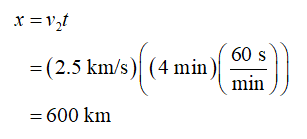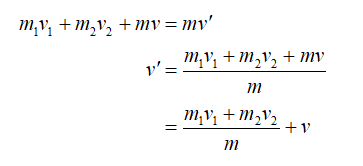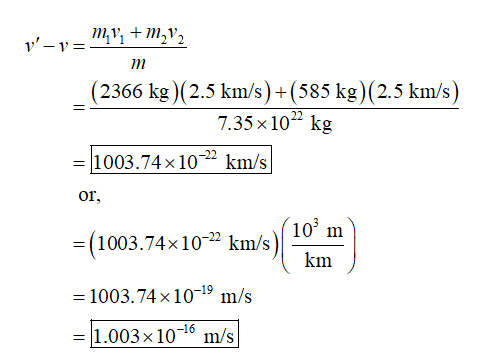# A spacecraft was following a rocket to the Moon. 4 minutes after the rocket impacted the Moon, the spacecraft itself crashed into the Moon. Both the Rocket and Spacecraft were moving at 2.5 km/s relative to the Moon when they impacted. The mass of the Rocket at the time of impact was 2366 kg, while the mass of the spacecraft was 585 kg.a) By what distance was the spacecraft following the Rocket?b) Determine the change in the velocity of the Moon due to the impact of the Rocket and spacecraft.c) How much more kinetic energy did the Rocket transfer to the Moon than the spacecraft transferred to the Moon?

Question
9 views

A spacecraft was following a rocket to the Moon. 4 minutes after the rocket impacted the Moon, the spacecraft itself crashed into the Moon. Both the Rocket and Spacecraft were moving at 2.5 km/s relative to the Moon when they impacted. The mass of the Rocket at the time of impact was 2366 kg, while the mass of the spacecraft was 585 kg.

a) By what distance was the spacecraft following the Rocket?

b) Determine the change in the velocity of the Moon due to the impact of the Rocket and spacecraft.

c) How much more kinetic energy did the Rocket transfer to the Moon than the spacecraft transferred to the Moon?

check_circle

Step 1

(a)

Let v1 be the velocity of the rocket, v2 be the velocity of spacecraft, m be the mass of the moon, v be the velocity of the moon before the impact, v’ be the velocity of the moon after the impact with the rocket and the spacecraft.

Let t be the time difference between the two impacts and x be the distance between the rocket and spacecraft.

Write the expression to determine the distance between the rocket and the spacecraft.Step 2

(b)

Write the expression for the conservation of momentum of the system at the time of impact of the rocket and moon.Step 3

Change in the velocity of the moon can be calculated as:...

### Want to see the full answer?

See Solution

#### Want to see this answer and more?

Solutions are written by subject experts who are available 24/7. Questions are typically answered within 1 hour.*

See Solution
*Response times may vary by subject and question.
Tagged in